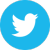Quantum Statistical Mechanics Mathematical Physics

A paper by Paula Cohen showing a surprising relationship between the Dedekind zeta function of a number field and a C*-dynamical system. PostScript.

Top: Science: Physics: Mathematical Physics: Statistical Mechanics: Quantum